Next: SLA_SVDCOV - Covariance Matrix from SVD
Up: SUBPROGRAM SPECIFICATIONS
Previous: SLA_SUPGAL - Supergalactic to Galactic

## SLA_SVD - Singular Value Decomposition

ACTION:
Singular value decomposition. This routine expresses a given matrix A as the product of three matrices U, W, VT:

A = UWVT

where:

A 		 is any m (rows)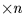(columns) matrix,                       where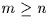U 		 is an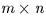column-orthogonal matrix
W 		 is an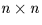diagonal matrix with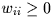VT is the transpose of anorthogonal matrix


CALL:
CALL sla_SVD (M, N, MP, NP, A, W, V, WORK, JSTAT)

GIVEN:

 I m, n, the numbers of rows and columns in matrix A M,N MP,NP I physical dimensions of array containing matrix A A D(MP,NP) array containingmatrix A

RETURNED:

 D(MP,NP) array containingcolumn-orthogonal matrix U A W D(N)diagonal matrix W (diagonal elements only) V D(NP,NP) array containingorthogonal matrix V (n.b. not VT) WORK D(N) workspace JSTAT I 0 = OK, -1 = array A wrong shape, >0 = index of W for which convergence failed (see note 3, below)

NOTES:
1.
M and N are the logical dimensions of the matrices and vectors concerned, which can be located in arrays of larger physical dimensions, given by MP and NP.
2.
V contains matrix V, not the transpose of matrix V.
3.
If the status JSTAT is greater than zero, this need not necessarily be treated as a failure. It means that, due to chance properties of the matrix A, the QR transformation phase of the routine did not fully converge in a predefined number of iterations, something that very seldom occurs. When this condition does arise, it is possible that the elements of the diagonal matrix W have not been correctly found. However, in practice the results are likely to be trustworthy. Applications should report the condition as a warning, but then proceed normally.

REFERENCES:
The algorithm is an adaptation of the routine SVD in the EISPACK library (Garbow et al. 1977, EISPACK Guide Extension, Springer Verlag), which is a FORTRAN 66 implementation of the Algol routine SVD of Wilkinson & Reinsch 1971 (Handbook for Automatic Computation, vol 2, ed Bauer et al., Springer Verlag). These references give full details of the algorithm used here. A good account of the use of SVD in least squares problems is given in Numerical Recipes (Press et al. 1987, Cambridge University Press), which includes another variant of the EISPACK code.Next: SLA_SVDCOV - Covariance Matrix from SVD
Up: SUBPROGRAM SPECIFICATIONS
Previous: SLA_SUPGAL - Supergalactic to Galactic

SLALIB --- Positional Astronomy Library